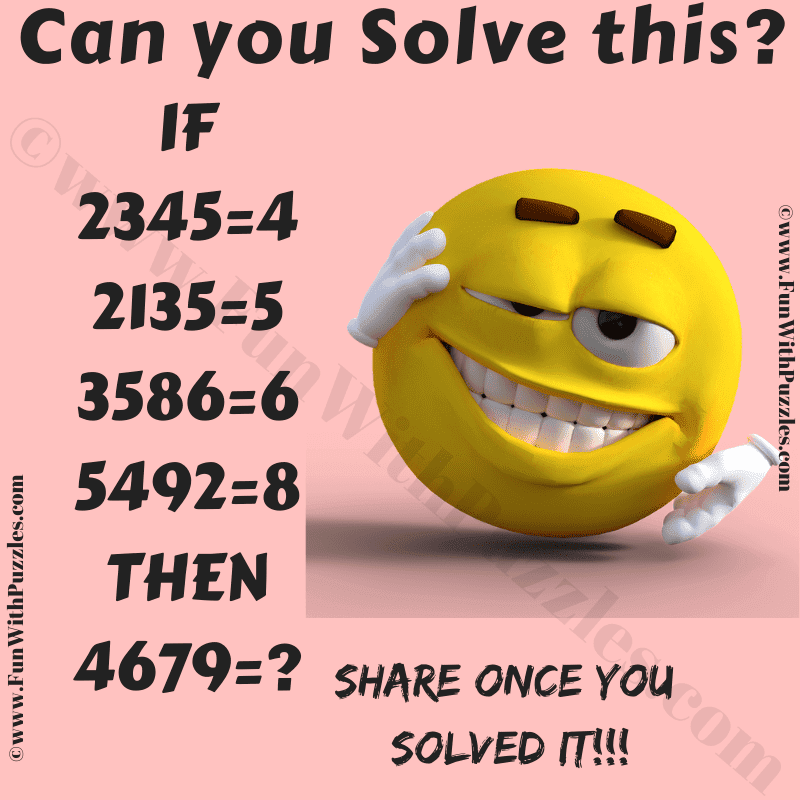This is a fun riddle that will test your logical and mathematical skills. Solving these kinds of maths logic riddles will help you to improve your logical and mathematical skills. In this puzzle, you are shown some logical number equations. Your challenge is to find the hidden logic in these equations and then solve the last equation. Let's see if you can crack the hidden code to find the missing number!Can you solve this Number Puzzle?

The answer to this "Fun Riddle with Maths and Logic", can be viewed by clicking on the answer button.